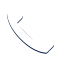Select Page

You can use a space heater to heat one room or part of your home during the winter, supplementing your central heating system and turning down the heat to save money. How much electricity does a space heater use? Read on to learn how to calculate the answer.

### Electricity Usage of Space Heaters

So how much electricity does a space heater use? Figure this out as follows:

1. Multiply the heater’s wattage by the hours the device is used in a day.
2. Divide the result by 1,000.
3. Multiply that answer by your electricity rate.

How much electricity does a space heater use? Most space heaters use 1,500 watts. Some use less or slightly more. Suppose you have a 1,500-watt heater. That means your heater uses 1.5 kilowatts of power (one kilowatt is equal to 1,000 watts).

Next, multiply the watts the heater uses by the hours you intend to use the device on average per day. Let’s assume you want to keep a room comfortable for reading for about eight hours. You’ll multiply 1,500 watts by 8 hours of use to get 12,000 watt-hours per day.

Your electricity bill is measured in kilowatt-hours (kWh). Therefore, you’ll convert watt-hours to kilowatt-hours by dividing 12,000 by 1,000 to get 12 kilowatt-hours. Then multiply the kilowatt-hours by your utility company’s electricity rate. For example, if your utility’s rate is \$0.28, you’ll multiply 12 by 0.28 to get \$3.36, meaning it might cost you \$3.36 per day to use your space heater. In other words, the answer to: How much energy does a space heater use?

When you’re in the market for a space heater, you can compare different models to get one that’s environmentally friendly. How much electricity does a space heater use? That’s an important question. Contact Mowery Heating, Cooling and Plumbing to learn about available heating and cooling options that will boost your comfort and energy efficiency.CALL MOWERY NOW